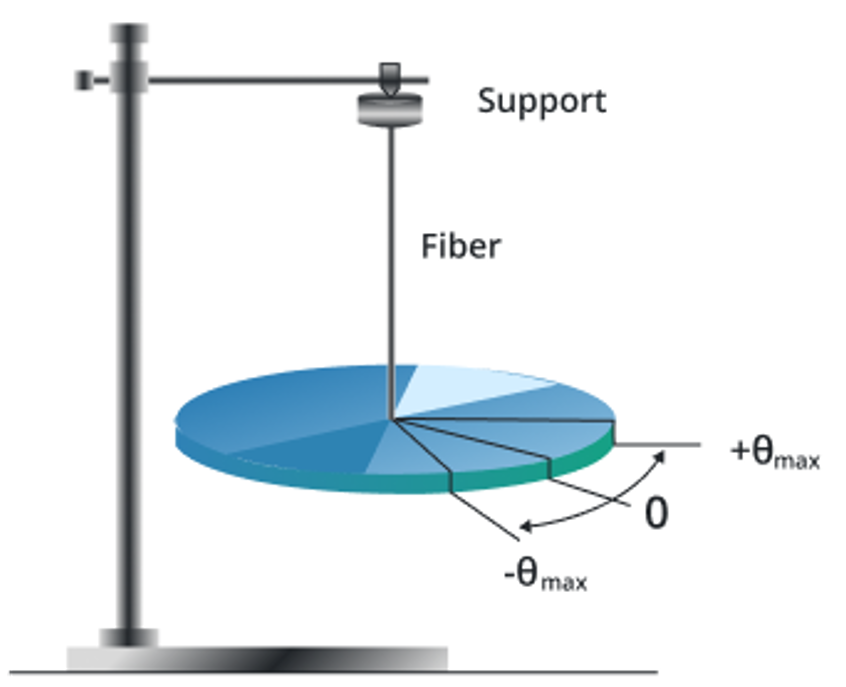# Torsional Pendulum Calculator

To find the time period of pendulum, enter the required values, and click calculate button

m
kgm2
Nm2

Give Us Feedback

## Torsional Pendulum Calculator

Torsional Pendulum Calculator is a mathematical tool that is used to find the time period of the pendulum by using the length of the spring and the moment of inertia of the spring that is suspended with a fixed position.

## What is meant by Torsional Pendulum?

A torsional pendulum is a mechanical system that consists of a spring-suspended to a fixed point from one end and attached a mass to the other end. It is a type of pendulum that oscillates in a rotational motion rather than the linear motion of a simple pendulum.## The formula of the torsional pendulum Time period

`Time Period (T) = 2π × √(I / (k × L²))`

Where,

• T = pendulum time period
• I = moment of inertia of the bob
• K = Torsional rigidity
• L = lengthy of the spring

## How to determine the Time period of the pendulum?

Example 1:

If the Pendulum length is 0.5 meters, the Moment of Inertia of the bob is 0.2 kilogram square meters and the Torsional Constant is 0.4 Newton.

Solution:

Step 1: Write the data from the question.

I = 0.2

K = 0.4

L = 0.5

T =?

Step 2: Write the formula of the torsional pendulum for a time period.

`T = 2π × √(I / (k × L²))`

Step 3: Put the values in the above formula.

T = 2π × √(0.2 / (0.4 × 0.5²))

T= 2π × √(0.2 / (0.1))

T= 2π × √(2)

`T = 8.88`

Example 2:

If the torsional Pendulum with a length is 4 meters, the Moment of Inertia of the bob is 128 kilogram square meters and the Torsional Constant is 2 Newton.

Solution:

Step 1: Write the data from the question.

I = 128

K = 2

L = 4

T =?

Step 2: Write the formula of the torsional pendulum for a time period.

`T = 2π × √(I / (k × L²))`

Step 3: Put the values in the above formula.

T= 2π × √(128 / (2 × 4²))

T= 2π × √(128/ (32))

T = 2π × √(4)

T = 2 × 3.14 × 2

`T = 12.56`

### Math Tools